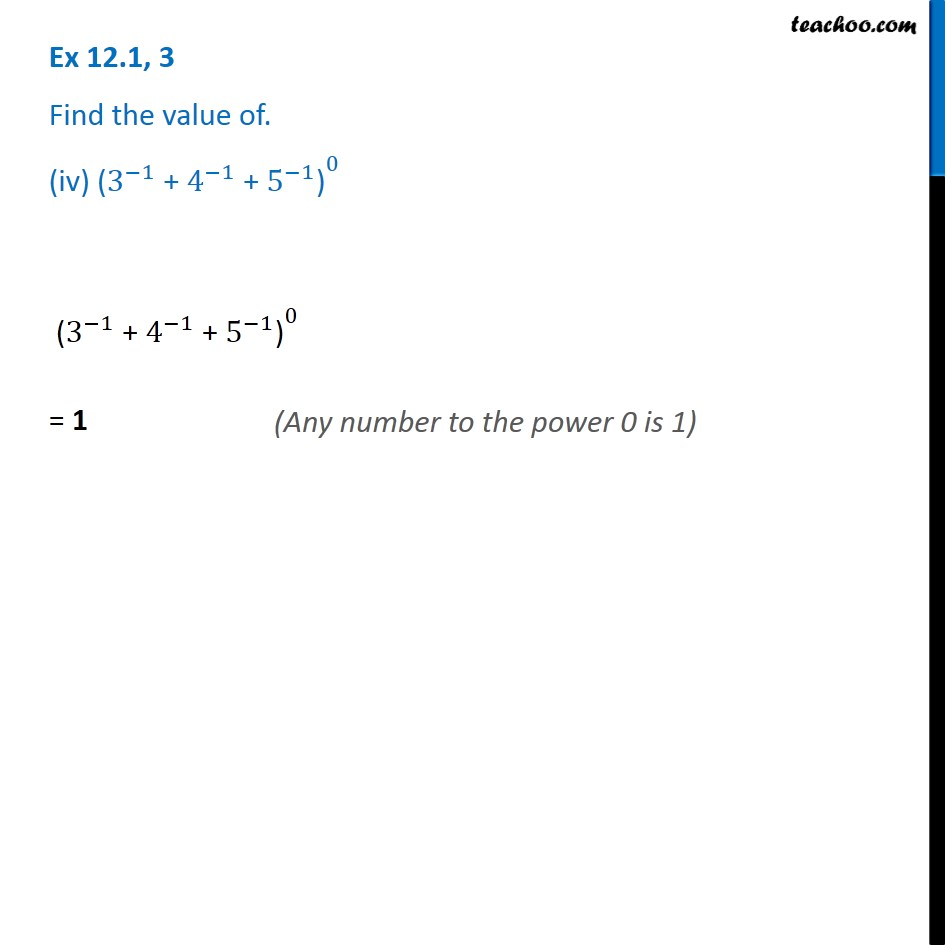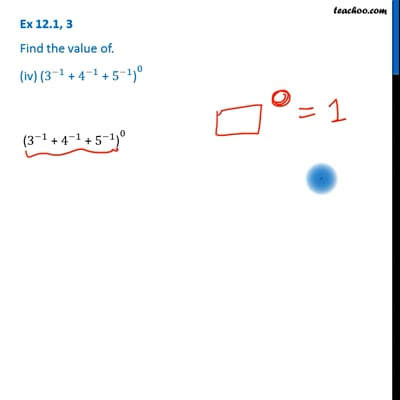Ex 12.1

Chapter 12 Class 8 Exponents and Powers
Serial order wiseThis video is only available for Teachoo black users

Get live Maths 1-on-1 Classs - Class 6 to 12

### Transcript

Ex 12.1, 3 Find the value of. (iv) 〖"(" 3^(−1) " + " 4^(−1) " + " 5^(−1) ")" 〗^0 〖"(" 3^(−1) " + " 4^(−1) " + " 5^(−1) ")" 〗^0 = 1 (Any number to the power 0 is 1)